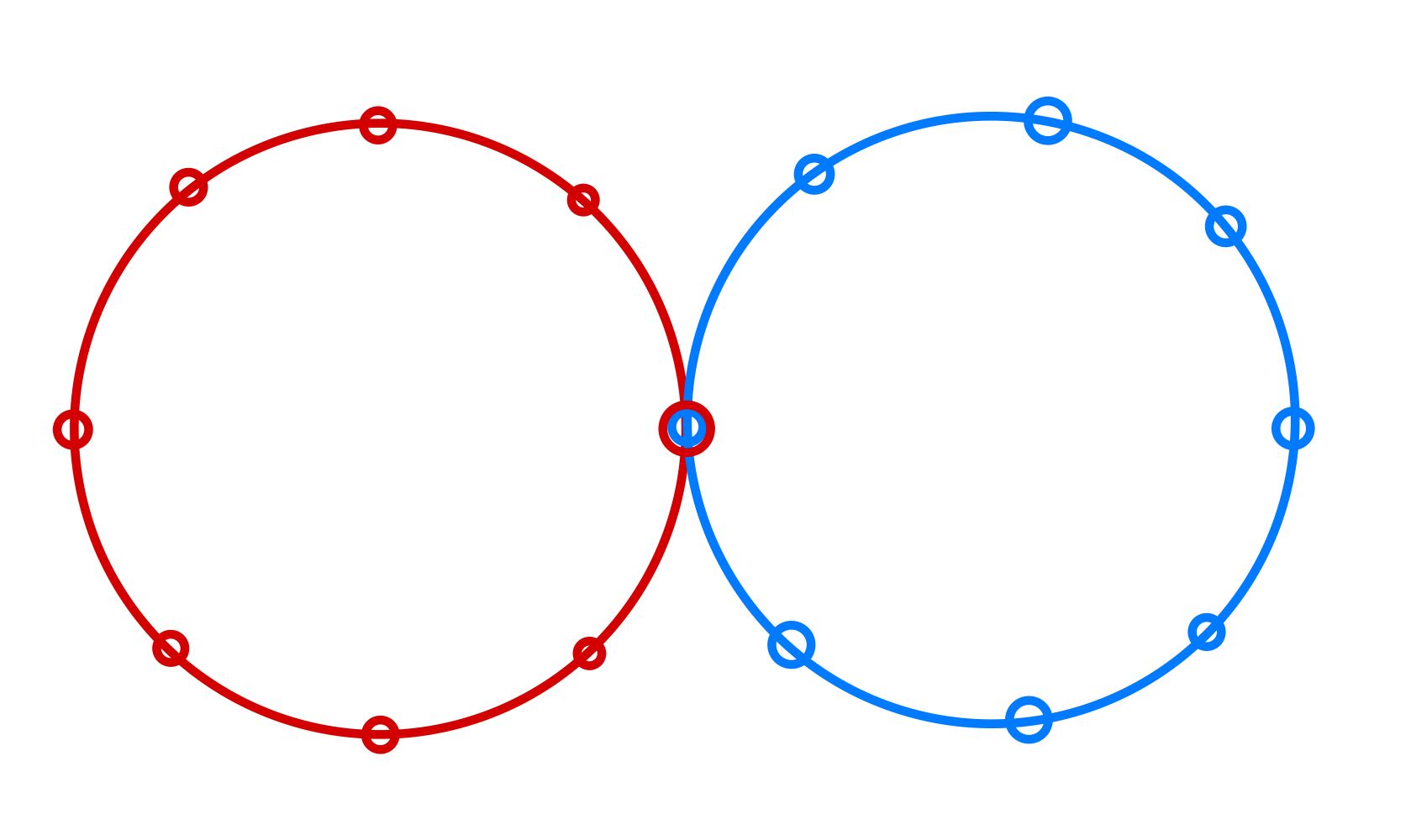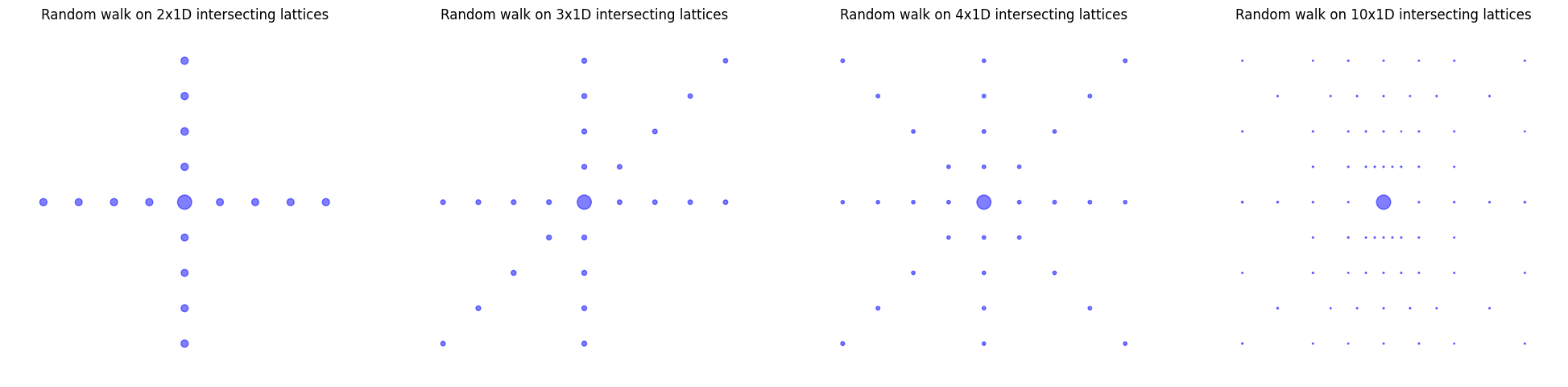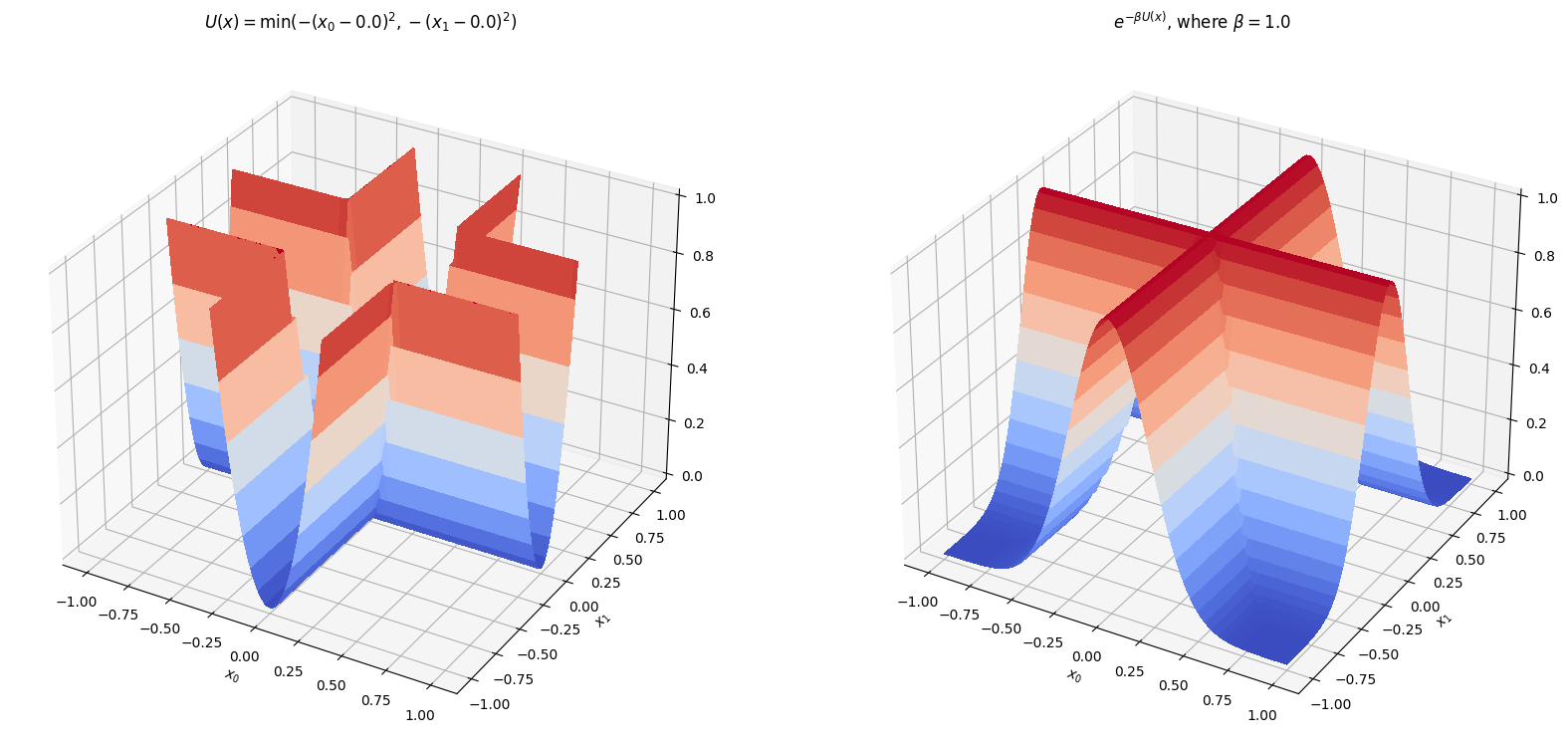# Ω 16

Crossposted from the AI Alignment Forum. May contain more technical jargon than usual.

Produced as part of the SERI ML Alignment Theory Scholars Program Winter 2022 Cohort.

Not all global minima of the (training) loss landscape are created equal.

Even if they achieve equal performance on the training set, different solutions can perform very differently on the test set or out-of-distribution. So why is it that we typically find "simple" solutions that generalize well?

In a previous post, I argued that the answer is "singularities" — minimum loss points with ill-defined tangents. It's the "nastiest" singularities that have the most outsized effect on learning and generalization in the limit of large data. These act as implicit regularizers that lower the effective dimensionality of the model.

Even after writing this introduction to "singular learning theory", I still find this claim weird and counterintuitive. How is it that the local geometry of a few isolated points determines the global expected behavior over all learning machines on the loss landscape? What explains the "spooky action at a distance" of singularities in the loss landscape?

Today, I'd like to share my best efforts at the hand-waving physics-y intuition behind this claim.

It boils down to this: singularities translate random motion at the bottom of loss basins into search for generalization.

# Random walks on the minimum-loss sets

Let's first look at the limit in which you've trained so long that we can treat the model as restricted to a set of fixed minimum loss points.

Here's the intuition pump: suppose you are a random walker living on some curve that has singularities (self-intersections, cusps, and the like). Every timestep, you take a step of a uniform length in a random available direction. Then, singularities act as a kind of "trap." If you're close to a singularity, you're more likely to take a step towards (and over) the singularity than to take a step away from the singularity.If we start at the blue point and uniformly sample the next location among the seven available locations for a fixed step size, we have 6:1 odds in favor of moving towards the singularity.

It's not quite an attractor (we're in a stochastic setting, where you can and will still break away every so often), but it's sticky enough that the "biggest" singularity will dominate your stable distribution.

In the discrete case, this is just the well-known phenomenon of high-degree nodes dominating most of expected behavior of your graph. In business, it's behind the reason that Google exists. In social networks, it's similar to how your average friend has more friends than you do.

To see this, consider a simple toy example: take two polygons and let them intersect at a single point. Next, let a random walker run loose on this setup. How frequently will the random walker cross each point?Take two or more 1D lattices with toroidal boundary conditions and let them intersect at one point. In the limit of an infinite polygon/lattice, you end up with a normal crossing singularity at the origin.

If you've taken a course in graph theory, you may remember that the equilibrium distribution weights nodes in proportion to their degrees. For two intersecting lines, the intersection is twice as likely as the other points. For three intersecting lines, it's three times as likely, and so on…The size of the circle shows how likely that point is under empirical simulation. The stationary distribution puts as many times more weight on the origin as there are intersecting lines.

Now just take the limit of infinitely large polygons/step size to zero, and we'll recover the continuous case we were originally interested in.

# Brownian motion near the minimum-loss set

Well, not quite. You see, restricting ourselves to motion along the minimum-loss points is unrealistic. We're more interested in messy reality, where we're allowed some freedom to bounce around the bottoms of loss basins.

This time around, the key intuition-pumping assumption is to view the behavior of stochastic gradient descent late in training as a kind of Brownian motion. When we've reached a low training-loss solution, variability between batches is a source of randomness that no longer substantially improves loss but just jiggles us between solutions that are equivalent from the perspective of the training set.

To understand these dynamics, we can just study the more abstract case of Brownian motion in some continuous energy landscape with singularities.

Consider the potential function given by

This is plotted on the left side of the following figure. The right side depicts the corresponding stable distribution as predicted by "regular" physics.An energy landscape whose minimum loss set has a normal crossing singularity at the origin. Toroidal boundary conditions as in the discrete case.

Simulating Brownian motion in this well generates an empirical distribution that looks rather different from the regular prediction…

As in the discrete case, the singularity at the origin gobbles up probability density, even at finite temperatures and even for points away from the minimum loss set.

# Takeaways

To summarize, the intuition is something like this: in the limiting case, we don't expect the model to learn much from any one additional sample. Instead, the randomness in drawing the new sample acts as Brownian motion that lets the model explore the minimum-loss set. Singularities are a trap for this Brownian motion which allow the model to find well-generalizing solutions just by moving around.

So SGD works because it ends up getting stuck near singularities, and singularities generalize further.

You can find the code for these simulations here and here.

EDIT: I found this post by @beren  that presents a very similar hypothesis.

1. ^

So technically, in singular learning theory we treat the loss landscape as changing with each additional sample. Here, we're considering the case that the landscape is frozen, and new samples act as a kind of random motion along the minimum-loss set.

2. ^

We're still treating the loss landscape as frozen but will now allow departures away from the minimum loss points.

3. ^

I.e.: the Gibbs measure.

4. ^

Let me emphasize: this is hand-waving/qualitative/physics-y jabber. Don't take it too seriously as a model for what SGD is actually doing. The "proper" way to think about this (thanks Dan) is in terms of the density of states.

# Ω 16

Mentioned in
New Comment

Epistemic status: Highly speculative hypothesis generation.

I had a similar, but slightly different picture. My picture was the tentacle covered blob.

When doing gradient descent from a random point, you usually get to a tentacle. (Ie the red trajectory)

When the system has been running for a while, it is randomly sampling from the black region. Most of the volume is in the blob. (The randomly wandering particle can easily find its way from the tentacle to the blob, but the chance of it randomly finding the end of a tentacle from within the blob is small)

Under this model, the generalization would work the same when using a valid sampling process.

When you start to include broad basins (where a small perturbation changes your loss but only slightly), and other results like Mingard et al.'s and Valle Pérez et al.'s, I'm inclined to think this picture is more representative. But even in this picture, we can probably end up making statements about singularities in the blobby regions having disproportionate effect over all points within those regions.

Instead of simulating Brownian motion, you could run SGD with momentum. That would be closer to what actually happens with NNs, and just as easy to simulate.

I expect it to be directionally similar but less pronounced (because MCMC methods with momentum explore the distribution better).

I also take issue with the way the conclusion is phrased. "Singularities work because they transform random motion into useful search for generalization". This is only true if you assume that points nearer a singularity generalize better. Maybe I'd phrase it as, "SGD works because it's more likely to end up near a singularity than the potential alone would predict, and singularities generalize better (see my [Jesse's] other post)". Would you agree with this phrasing?

Instead of simulating Brownian motion, you could run SGD with momentum. That would be closer to what actually happens with NNs, and just as easy to simulate.

Hey I need a reason to write a follow-up to this, right?

I also take issue with the way the conclusion is phrased. "Singularities work because they transform random motion into useful search for generalization". This is only true if you assume that points nearer a singularity generalize better. Maybe I'd phrase it as, "SGD works because it's more likely to end up near a singularity than the potential alone would predict, and singularities generalize better (see my [Jesse's] other post)". Would you agree with this phrasing?

I was trying to be intentionally provocative, but you're right — it's too much. Thanks for the suggestion!